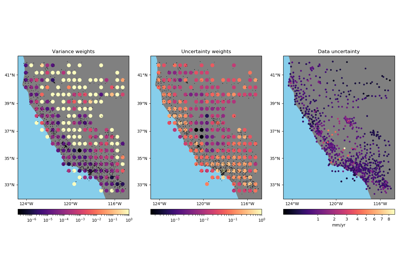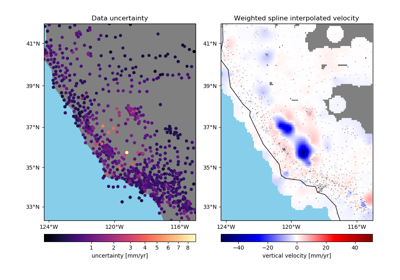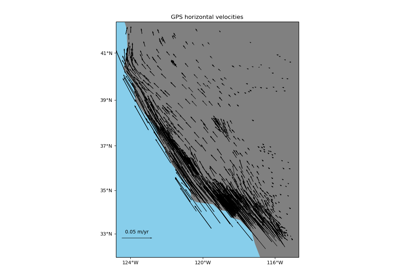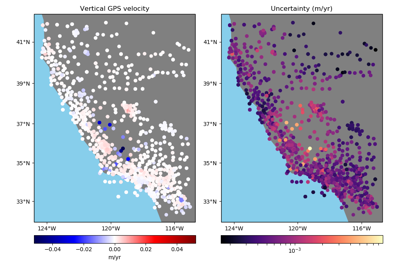# verde.BlockMean¶

class verde.BlockMean(spacing, region=None, adjust='spacing', center_coordinates=False, uncertainty=False)[source]

Apply a (weighted) mean to the data in blocks/windows.

Returns the mean data value for each block along with the associated coordinates and weights. Coordinates can be determined through the mean of the data coordinates or as the center of each block. Weights can be calculated in three ways:

1. Using the variance of the data: weights=1/variance. This is the only possible option when no input weights are provided.
2. Using the uncertainty of the weighted mean propagated from the uncertainties in the data: weights=1/uncertainty**2. In this case, we assume that the input weights are also 1/uncertainty**2. Do not normalize or scale the weights if using uncertainty propagation.
3. Using the weighted variance of the data: 1/weighted_variance. In this case, we make no assumptions about the nature of the weights.

For all three options, the output weights are scaled to the range [0, 1].

This class always outputs weights. If you want to calculate a blocked mean and not output any weights, use verde.BlockReduce with numpy.average instead.

Using the propagated uncertainties may be more adequate if your data is smooth in each block but have very different uncertainties. The propagation will preserve a low weight for data that have large uncertainties but don’t vary much inside the block.

The weighted variance should be used when the data vary a lot in each block but have very similar uncertainties. This is also the best choice if your input weights aren’t 1/uncertainty**2 but are a relative importance of the data instead.

If a data region to be divided into blocks is not given, it will be the bounding region of the data. When using this class to decimate data before gridding, it’s best to use the same region and spacing as the desired grid.

If the given region is not divisible by the spacing (block size), either the region or the spacing will have to be adjusted. By default, the spacing will be rounded to the nearest multiple. Optionally, the East and North boundaries of the region can be adjusted to fit the exact spacing given.

Blocks without any data are omitted from the output.

Implements the filter method so it can be used with verde.Chain. Only acts during data fitting and is ignored during prediction.

Parameters: spacing : float, tuple = (s_north, s_east), or None The block size in the South-North and West-East directions, respectively. A single value means that the size is equal in both directions. region : list = [W, E, S, N] The boundaries of a given region in Cartesian or geographic coordinates. adjust : {‘spacing’, ‘region’} Whether to adjust the spacing or the region if required. Ignored if shape is given instead of spacing. Defaults to adjusting the spacing. center_coordinates : bool If True, then the returned coordinates correspond to the center of each block. Otherwise, the coordinates are calculated by applying the same reduction operation to the input coordinates. uncertainty : bool If True, the blocked weights will be calculated by uncertainty propagation of the data uncertainties. If this is case, then the input weights must be 1/uncertainty**2. Do not normalize the input weights. If False, then the blocked weights will be calculated as 1/variance and no assumptions are made of the input weights (so they can be normalized).

block_split
Split a region into blocks and label points accordingly.
BlockReduce
Apply the mean in blocks. Will output weights.
verde.Chain
Apply filter operations successively on data.

Methods

 filter(coordinates, data[, weights]) Apply the blocked mean to the given data. get_params([deep]) Get parameters for this estimator. set_params(**params) Set the parameters of this estimator.

## Examples using verde.BlockMean¶Using weights in blocked meansGridding with splines and weightsVector DataUsing Weights

BlockMean.filter(coordinates, data, weights=None)[source]

Apply the blocked mean to the given data.

Returns the reduced data value for each block along with the associated coordinates and weights. See the class docstring for details.

Parameters: coordinates : tuple of arrays Arrays with the coordinates of each data point. Should be in the following order: (easting, northing, vertical, …). Only easting and northing will be used, all subsequent coordinates will be ignored. data : array or tuple of arrays The data values at each point. If you want to reduce more than one data component, pass in multiple arrays as elements of a tuple. All arrays must have the same shape. weights : None or array or tuple of arrays If not None, then the weights assigned to each data point. If more than one data component is provided, you must provide a weights array for each data component (if not None). If calculating the output weights through uncertainty propagation, then weights must be 1/uncertainty**2. blocked_coordinates : tuple of arrays (easting, northing) arrays with the coordinates of each block that contains data. blocked_mean : array or tuple of arrays The block averaged data values. blocked_weights : array or tuple of arrays The weights calculated for the blocked data values.
BlockMean.get_params(deep=True)

Get parameters for this estimator.

Parameters: deep : boolean, optional If True, will return the parameters for this estimator and contained subobjects that are estimators. params : mapping of string to any Parameter names mapped to their values.
BlockMean.set_params(**params)

Set the parameters of this estimator.

The method works on simple estimators as well as on nested objects (such as pipelines). The latter have parameters of the form <component>__<parameter> so that it’s possible to update each component of a nested object.

Returns: self